# MCQ Questions for Class 9 Maths Chapter 6 Lines and Angles with Answers

Check the below NCERT MCQ Questions for Class 9 Maths Chapter 6 Lines and Angles with Answers Pdf free download. MCQ Questions for Class 9 Maths with Answers were prepared based on the latest exam pattern. We have provided Lines and Angles Class 10 Maths MCQs Questions with Answers to help students understand the concept very well. https://mcqquestions.guru/mcq-questions-for-class-9-maths-chapter-6/

Students can also refer to NCERT Solutions for Class 9 Maths Chapter 6 Lines and Angles for better exam preparation and score more marks.

## Lines and Angles Class 9 MCQs Questions with Answers

Lines And Angles Class 9 MCQ Question 1.
In ΔABC, ∠A = 50° and the external bisectors of ∠B and ∠C meet at O as shown in figure. The measure of ∠BOC is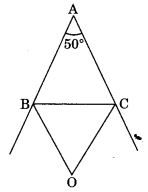(a) 40°
(b) 65°
(c) 115°
(d) 140°

Class 9 Maths Chapter 6 MCQ Question 2.
In figure the value of x is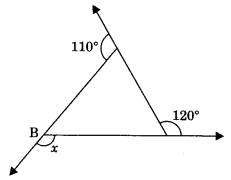(a) 120°
(b) 130°
(c) 110°
(d) 100°

Class 9 Lines And Angles MCQ Question 3.
An exterior angle of a triangle is 80° and the interior opposite angles are in the ratio 1 : 3, measure of interior opposite angles are
(a) 30°, 90°
(b) 40°, 120°
(c) 20°, 60°
(d) 30°, 60°

Lines And Angles MCQ Class 9 Question 4.
In ΔABC, the bisectors of ∠ABC and ∠BCA intersect each other at O. The measure of ∠BOC is
(a) 90° + ∠A
(b) 90° + $$\frac{∠A}{2}$$
(c) 180 – ∠A
(d) 90° – $$\frac{∠A}{2}$$

Answer: (b) 90° + $$\frac{∠A}{2}$$

MCQ Of Lines And Angles Class 9 Question 5.
In figure if ∠A + ∠B + ∠C + ∠D + ∠E + ∠F = k right angles, then find value of k.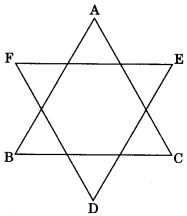(а) 2
(b) 3
(c) 4
(d) 5

Ch 6 Maths Class 9 MCQ Question 6.
In the given figure, the measure of ∠ABC is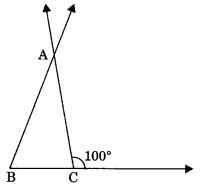(a) 80°
(b) 20°
(c) 100°
(d) 60°

MCQ On Lines And Angles Class 9 Question 7.
The angle of a triangle are in the ratio 5 : 3 : 7, the triangle is
(а) an acute-angled triangle
(b) an obtuse angled triangle
(c) an right angled triangle
(d) an isosceles triangle.

Class 9 Maths Ch 6 MCQ Question 8.
In figure l1 || l2, the value of x is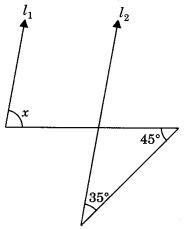(a) 80°
(b) 100°
(c) 110°
(d) 70°

Class 9 Maths Lines And Angles MCQ Question 9.
If one angle of triangle is equal to the sum of the other two, then the triangle is
(a) an isosceles triangle
(b) an obtuse-angled triangle
(c) an equilateral triangle
(d) a right triangle

Chapter 6 Maths Class 9 MCQ Question 10.
One of the angles of a triangle is 75°. If the difference of other two is 35°, then the largest angle of other two angles has a measure
(a) 80°
(b) 75°
(c) 70°
(d) 135°

Lines And Angles Class 9 MCQ With Answers Question 11.
An exterior angle of a triangle is 105° and its two interior opposite angles are equal. Each of these equal angles is
(a) 37 $$\frac{1°}{2}$$
(b) 52 $$\frac{1°}{2}$$
(c) 72 $$\frac{1°}{2}$$
(d) 75°

Answer: (b) 52 $$\frac{1°}{2}$$

MCQ Lines And Angles Class 9 Question 12.
In Figure measure of ∠ABC is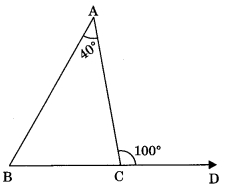(a) 60°
(b) 70°
(c) 80°
(d) 50°

Maths Class 9 Chapter 6 MCQ Question 13.
In Figure AB || CD, the value of x is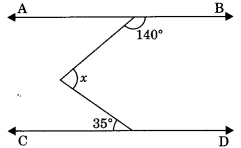(a) 35°
(b) 40°
(c) 60°
(d) 75°

Lines And Angles Class 9 MCQ With Answers Pdf Question 14.
In the figure, PS ⊥ l, RQ ⊥ l, the degree measure of y is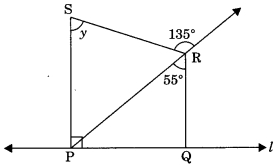(a) 55°
(b) 90°
(c) 80°
(d) 135°

Class 9 Ch 6 Maths MCQ Question 15.
Each angle of an equilateral triangle is
(a) 50°
(b) 90°
(c) 54°
(d) 60°

MCQ Questions For Class 9 Maths Lines And Angles Question 16.
Given lines l1 || l2 and l2 || l3 in figure. The value of x is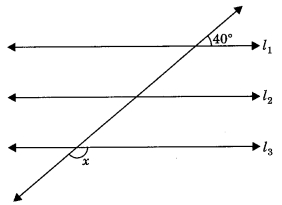(a) 40°
(b) 140°
(c) 50°
(d) 80°

Class 9 Chapter 6 Maths MCQ Question 17.
In figure, find the value of y.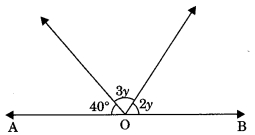(a) 28°
(b) 40°
(c) 140°
(d) 56°

Class 9th Maths Chapter 6 MCQ Question 18.
In the given figure, if AOB is a straight line, then ∠BOC is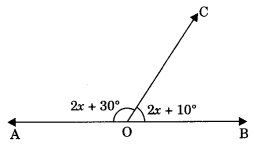(a) 80°
(b) 70°
(c) 60°
(d) 20°